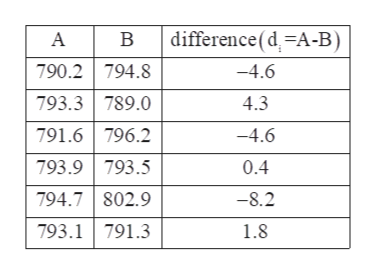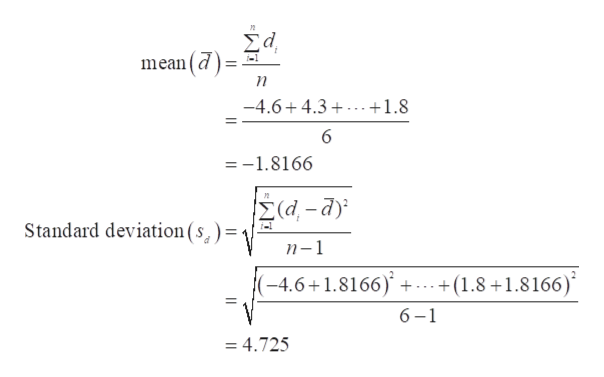# The following data represent the muzzle velocity​ (in feet per​ second) of rounds fired from a​ 155-mm gun. For each​ round, two measurements of the velocity were recorded using two different measuring​ devices, resulting in the following data. Complete parts​ (a) through​ (d) below.Observation123456 A790.2790.2793.3793.3791.6791.6793.9793.9794.7794.7793.1793.1 B794.8794.8789.0789.0796.2796.2793.5793.5802.9802.9791.3791.3 ​(a) Why are these​ matched-pairs data?A.All the measurements came from rounds fired from the same gun.B.Two measurements​ (A and​ B) are taken on the same round.Your answer is correct.C.The measurements​ (A and​ B) are taken by the same instrument.D.The same round was fired in every trial.​(b) Is there a difference in the measurement of the muzzle velocity between device A and device B at thealpha equals 0.01α=0.01 level of​ significance? ​Note: A normal probability plot and boxplot of the data indicate that the differences are approximately normally distributed with no outliers.Let diequals=Aiminus−Bi. Identify the null and alternative hypotheses.Upper H 0H0​:mu Subscript dμdequals=00Upper H 1H1​:mu Subscript dμdnot equals≠00Determine the test statistic for this hypothesis test.t0equals ​(Round to two decimal places as​ needed.)Find the​ P-value.​P-valueequals=nothing​(Round to three decimal places as​ needed.)

Question
120 views

The following data represent the muzzle velocity​ (in feet per​ second) of rounds fired from a​ 155-mm gun. For each​ round, two measurements of the velocity were recorded using two different measuring​ devices, resulting in the following data. Complete parts​ (a) through​ (d) below.

 Observation 1 2 3 4 5 6 A 790.2 790.2 793.3 793.3 791.6 791.6 793.9 793.9 794.7 794.7 793.1 793.1 B 794.8 794.8 789.0 789.0 796.2 796.2 793.5 793.5 802.9 802.9 791.3 791.3

​(a) Why are these​ matched-pairs data?

A.

All the measurements came from rounds fired from the same gun.

B.

Two measurements​ (A and​ B) are taken on the same round.

C.

The measurements​ (A and​ B) are taken by the same instrument.

D.

The same round was fired in every trial.

​(b) Is there a difference in the measurement of the muzzle velocity between device A and device B at the

alpha equals 0.01

α=0.01 level of​ significance? ​Note: A normal probability plot and boxplot of the data indicate that the differences are approximately normally distributed with no outliers.

Let di

equals

=Ai

minus

−Bi. Identify the null and alternative hypotheses.

Upper H 0

H0​:

mu Subscript d

μd

equals

=

0

0

Upper H 1

H1​:

mu Subscript d

μd

not equals

0

0

Determine the test statistic for this hypothesis test.

t0

equals

​(Round to two decimal places as​ needed.)

Find the​ P-value.

​P-value

equals

=

nothing

​(Round to three decimal places as​ needed.)

check_circle

Step 1

a.

From the provided information, the two measurements (A and B) are taken on the same round. Therefore, these are matched pair data.

Thus, option B) is correct.

b.

The difference table between the data can be obtained as:help_outlineImage Transcriptionclosedifference (d-A-B В 790.2 794.8 -4.6 793.3 789.0 4.3 791.6 796.2 793.9793.5 -4.6 0.4 794.7 802.9 -8.2 793.1 791.3 1.8 fullscreen
Step 2

The mean and standard deviation of difference can be obtained as:help_outlineImage TranscriptioncloseΣd mean(d -4.6+4.3 +1.8 6 =-1.8166 (d-a) Standard deviation (s) = n-1 (-4.6+1.8166)' + .+(1.8+1.8166) 6-1 = 4.725 fullscreen
Step 3

The null and alternate hypothesis can be constructed as:

Null hypothesis, H0:µd=0. (There is no significance difference in the measurement of velocity.)

Alternative hypothesis, H1:µ...

### Want to see the full answer?

See Solution

#### Want to see this answer and more?

Solutions are written by subject experts who are available 24/7. Questions are typically answered within 1 hour.*

See Solution
*Response times may vary by subject and question.
Tagged in

### Statistics